Question

# A single mass m1 = 3.5 kg hangs from a spring in a motionless elevator. The...

A single mass m1 = 3.5 kg hangs from a spring in a motionless elevator. The spring is extended x = 15 cm from its unstretched length.

1) What is the spring constant of the spring?

Now, three masses m1 = 3.5 kg, m2 = 10.5 kg and m3 = 7 kg hang from three identical springs in a motionless elevator. The springs all have the same spring constant that you just calculated above.

What is the force the top spring exerts on the top mass?

3) What is the distance the lower spring is stretched from its equilibrium length?

4) Now the elevator is moving downward with a velocity of v = -2.9 m/s but accelerating upward with an acceleration of a = 3.7 m/s2. (Note: an upward acceleration when the elevator is moving down means the elevator is slowing down.)

What is the force the bottom spring exerts on the bottom mass?

5) What is the distance the upper spring is extended from its unstretched length?

8) What is the distance the MIDDLE spring is extended from its unstretched length?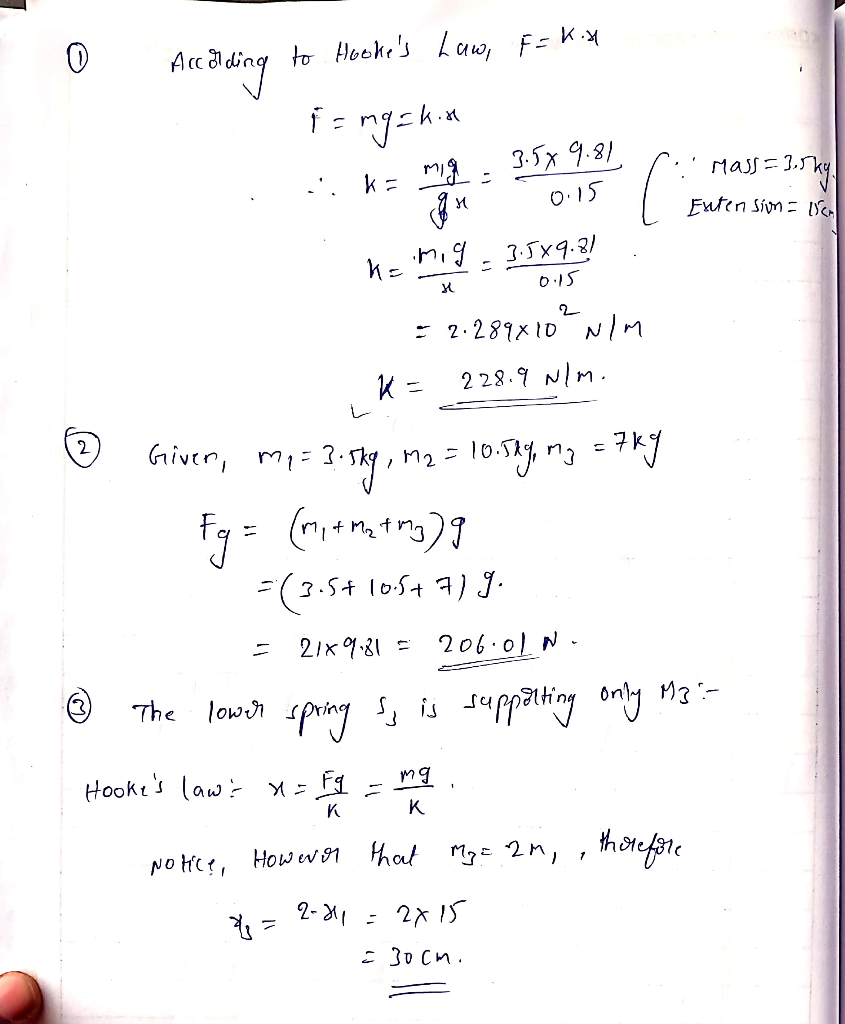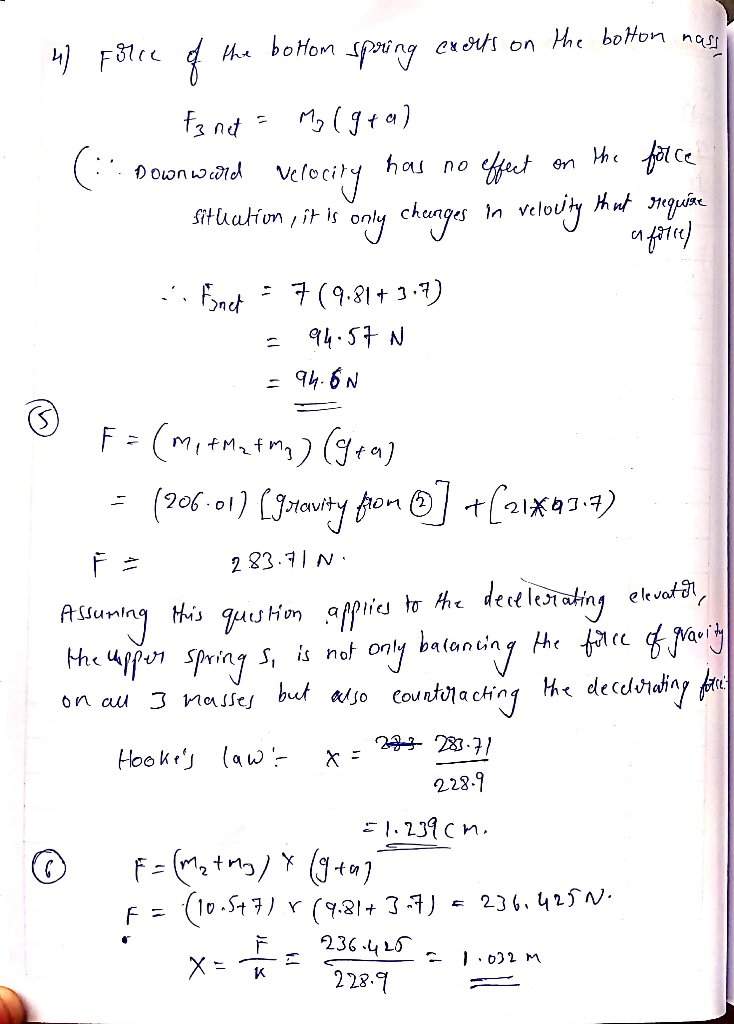#### Earn Coins

Coins can be redeemed for fabulous gifts.

Similar Homework Help Questions
• ### A single mass (m1 = 4.4 kg) hangs from a spring in a motionless elevator.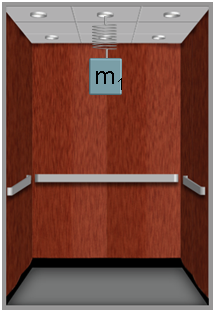A single mass (m1 = 4.4 kg) hangs from a spring in a motionless elevator. The spring constant is k = 270 N/m. 1) What is the distance the spring is stretched from its unstretched length?  2) Now, three masses (m1 = 4.4 kg, m2 = 13.2 kg and m3 = 8.8) hang from three identical springs in a motionless elevator. The springs all have the same spring constant given above. What is the magnitude of the force the bottom spring exerts on the...

• ### A single mass m1 = 4.4 kg hangs from a spring in a motionless elevator. The...

A single mass m1 = 4.4 kg hangs from a spring in a motionless elevator. The spring is extended x = 14 cm from its unstretched length. Now, three masses m1 = 4.4 kg, m2 = 13.2 kg and m3 = 8.8 kg hang from three identical springs in a motionless elevator. The springs all have the same spring constant that you just calculated above. 1) What is the force the top spring exerts on the top mass? 2) What...

• ### I need the answers for # 5 and #8 2) m m mi Now, three masses...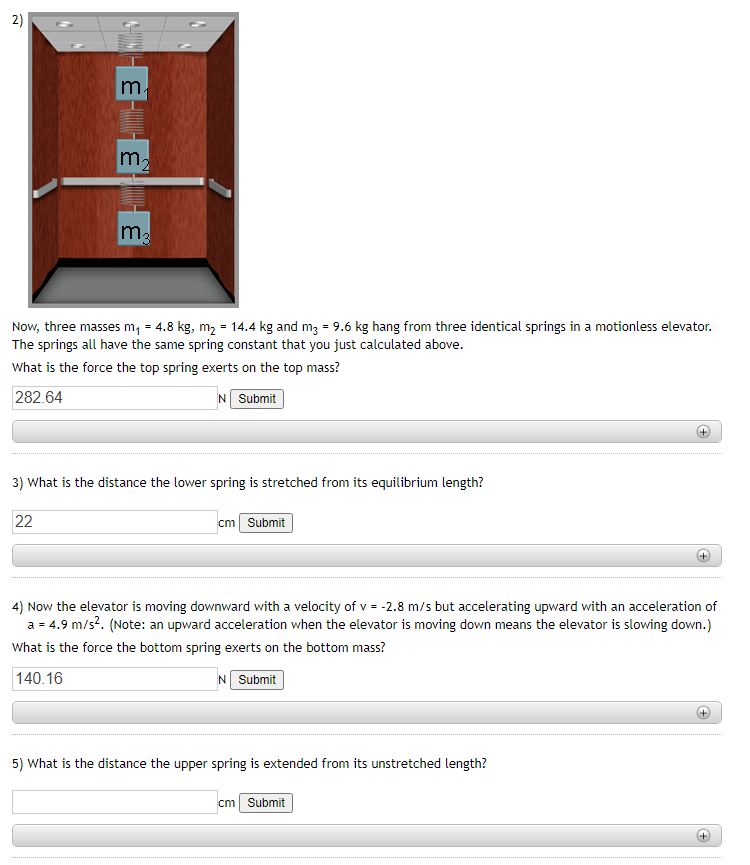I need the answers for # 5 and #8 2) m m mi Now, three masses m, = 4.8 kg, m2 = 14.4 kg and m2 = 9.6 kg hang from three identical springs in a motionless elevator. The springs all have the same spring constant that you just calculated above. What is the force the top spring exerts on the top mass? 282.64 N Submit + 3) What is the distance the lower spring is stretched from its equilibrium...

• ### Now, three masses m1=3.4kg, m2=10.2kg and m3=6.8kg x=14 hang from three identical springs in a motionless...

Now, three masses m1=3.4kg, m2=10.2kg and m3=6.8kg x=14 hang from three identical springs in a motionless elevator. Finally the elevator is moving downward with a velocity of v=-2.8m/s and also accelerating downward at an acceleration of a=-3.3m/s^2. The elevator is A. speeding up B. slowing down C. moving at a constant speed Rank the distances the springs are extended from their unstretched lengths: A. x1=x2=X3 B.X1>x2>x3 C. x1<x2<x3 what is the distance the Middle spring is extended from its unstretched...

• ### I need answers for #5 and #7 2) m m2 ma Now, three masses (m, =...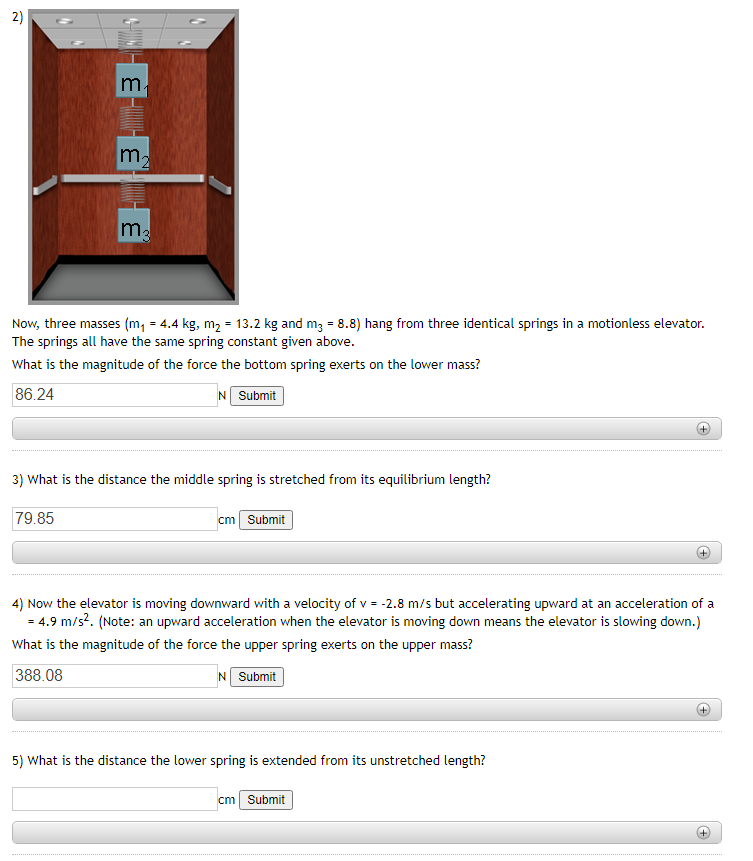I need answers for #5 and #7 2) m m2 ma Now, three masses (m, = 4.4 kg, m2 = 13.2 kg and mz = 8.8) hang from three identical springs in a motionless elevator. The springs all have the same spring constant given above. What is the magnitude of the force the bottom spring exerts on the lower mass? 86.24 N Submit + 3) What is the distance the middle spring is stretched from its equilibrium length? 79.85 cm...

• ### A mass m1=5.0 kg hangs suspended by a rope from an elevator ceiling. A second mass...

A mass m1=5.0 kg hangs suspended by a rope from an elevator ceiling. A second mass m2=5.0 kg hangs suspended by a rope from the bottom of mass m1. Starting from rest, the elevator ascends, attaining its maximum speed of 1.8 m/s in 0.80 s. The elevator travels with this constant speed for 5.0 s, undergoes a uniform negative acceleration for 1.5 s, and then comes to rest. Draw a FBD for each mass Find the tension in each rope...

• ### A block with mass m1 = 8.5 kg is on an incline with an angle θ...

A block with mass m1 = 8.5 kg is on an incline with an angle θ = 25° with respect to the horizontal. For the first question there is no friction between the incline and the block. 1)When there is no friction, what is the magnitude of the acceleration of the block? 2)Now with friction, the acceleration is measured to be only a = 2.74 m/s2. What is the coefficient of kinetic friction between the incline and the block? 3)...

• ### 5. A passenger of mass 85 kg is riding in an elevator while The scale only reads the normal force it exerts on the (Note that 1 b 0.453 kg 4.45 N) a) What standing on a platform scale. does the s...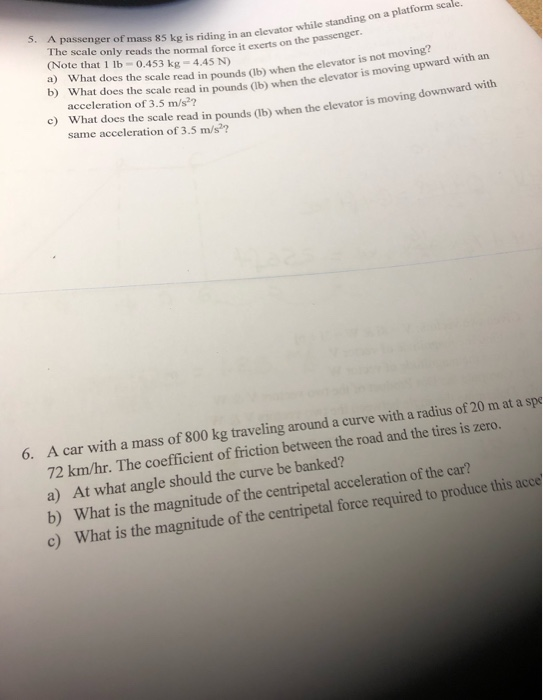5. A passenger of mass 85 kg is riding in an elevator while The scale only reads the normal force it exerts on the (Note that 1 b 0.453 kg 4.45 N) a) What standing on a platform scale. does the scale read in pounds (lb) when the elevator is not moving? elevator is moving upward with an acceleration of 3.5 m/s?? c) What does the scale read in pounds (b) when the elevator is same acceleration of 3.5 m/s??...

• ### A block with mass m1 = 8.9 kg is on an incline with an angle θ = 27°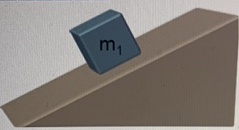A block with mass m1 = 8.9 kg is on an incline with an angle θ = 27° with respect to the horizontal. For the first question there is no friction between the incline and the block. 1) When there is no friction, what is the magnitude of the acceleration of the block? 2) Now with friction, the acceleration is measured to be only a= 3.38 m/s2. What is the coefficient of kinetic friction between the incline and the block? 3)  To keep the...

• ### m1 A block with mass my = 9.4 kg is on an incline with an angle...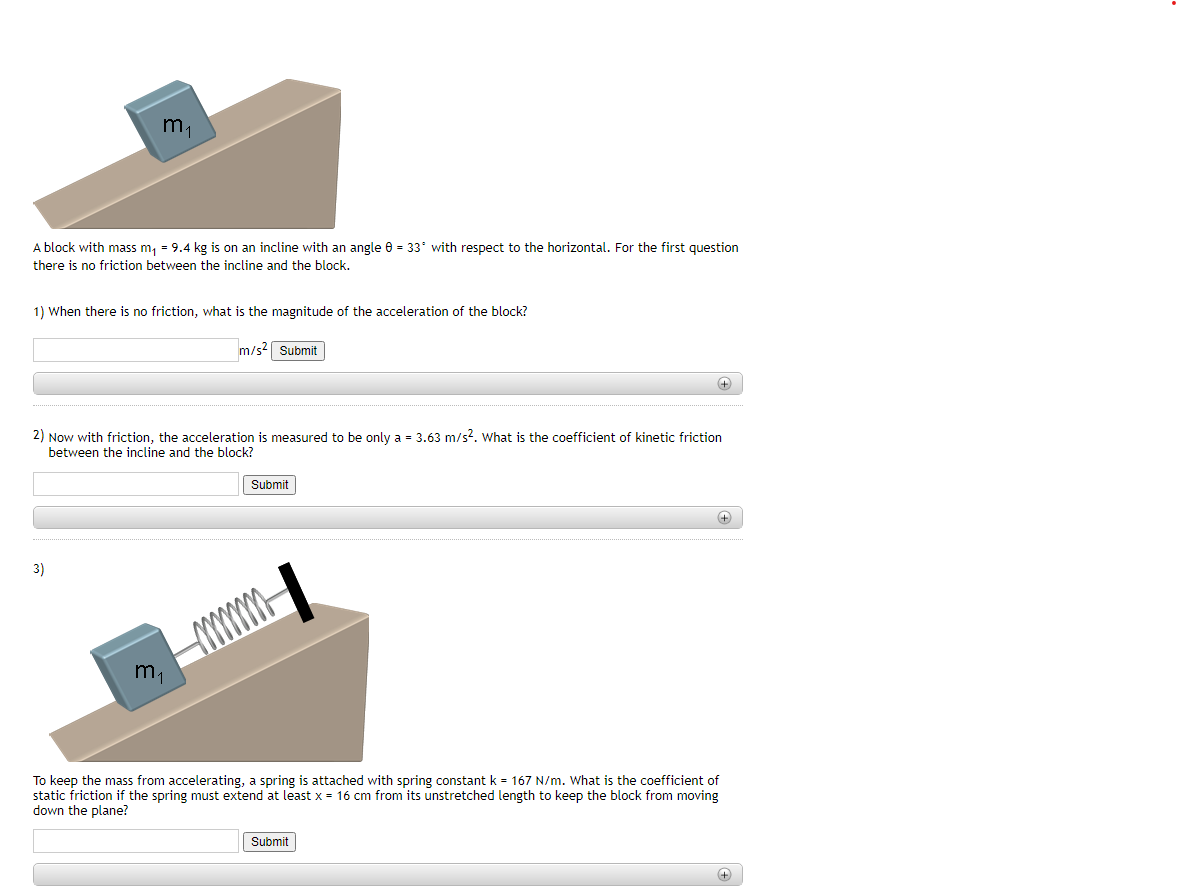m1 A block with mass my = 9.4 kg is on an incline with an angle 6 = 33 with respect to the horizontal. For the first question there is no friction between the incline and the block. 1) When there is no friction, what is the magnitude of the acceleration of the block? m/s2 Submit 2) Now with friction, the acceleration is measured to be only a = 3.63 m/s2. What is the coefficient of kinetic friction between the...